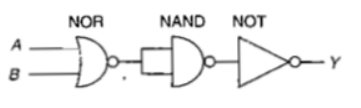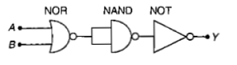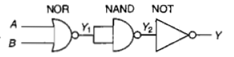The circuit is equivalent to(a) AND gate                              (b) NAND gate

(c) NOR gate                              (d) OR gate

The gate circuit can be shown by giving two inputs A and B.Output of NOR gate,

${Y}_{1}=\overline{A+B}$

Output of NAND gate,

${Y}_{2}=\overline{{Y}_{1}·{Y}_{1}}$

$=\overline{A+B·A+B}$

$=\overline{A+B}+\overline{A+B}$

$=A+B$

Output of NOT gate,

$Y=\overline{{Y}_{2}}$

$=\overline{A+B}$

which is the output of NOR gate.

Alternative:-NOR                                     NAND                             NOT

A             B             ${Y}_{1}$           ${Y}_{1}$            ${Y}_{1}$               ${Y}_{2}$             $Y$

0             0             1              1              1                 0               1

0             1             0              0              0                 1               0

1             0             0              0              0                 1               0

1             1             0              0              0                 1               0

Same as NOR Gate

NOR Gate

0                0               1

0                1               0

1                0               0

1                1               0

Note : NOR and NAND gates are said to be universal building blocks.

Difficulty Level:

• 13%
• 25%
• 51%
• 13%